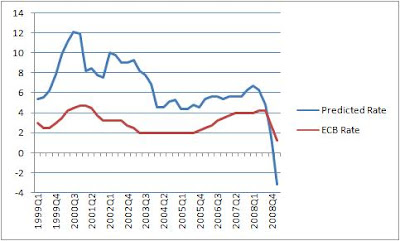## Tuesday, April 28, 2009

### Not really Taylored to suit

Setting interest rates involves a balancing act between "price stability" and "economic growth". In an influential 1993 paper "Discretion Versus Policy Rules in Practice" Stanford professor John Taylor proposed the following rule for setting the central bank interest rate:

Interest Rate = Inflation + 2.0 + 0.5 (Inflation − 2.0)
+ 0.5 (GDP gap).

The interest rate is the policy rate set by the monetary authority or central bank. Inflation is a measure of domestic price changes and the GDP gap is the percentage by which real GDP deviates from an estimate of its natural level.

According to this rule, the policy interest rate equals 4 percent when inflation is 2 percent and GDP is at its natural level. The first constant of 2 percent in the equation can be interpreted as an estimate of the natural rate of interest, and the second constant of 2 percent subtracted from inflation can be interpreted as the inflation target.

For each percentage point that inflation rises above 2 percent, the interest rate rises by 0.5 percent. For each percentage point that real GDP rises above its natural level, the rate rises by 0.5 percent. If inflation falls below 2 percent or GDP moves below its natural level, the interest rate falls accordingly.

In 1999 Ireland handed control of our monetary policy to the ECB meaning that our interest rate is set in Frankfurt rather than Dublin. What would we see if we compare the eurozone interest rate as set by the ECB to the predicted rate of the Taylor rule if we applied it to crude Irish data for the past decade?

In the following graph inflation is measured using the Harmonised Index of Consumer Prices (HICP) as used by the ECB and the GDP gap is measured as the difference between the Non Accelerating Inflation Rate of Unemployment (NAIRU) as produced by the OCED and the actual unemployment rate. All data are quarterly.The result is pretty clear. For the last ten years interest rates have been too low in Ireland. The predicted rate from the Taylor rule has been an average of three and a half percent higher than the rate applied by the ECB. The rule suggests that rates should never have been set below four percent and that during the "peak" of the boom they could have been as high as 12 percent. The interest rates set by the ECB were too low and no doubt contributed to the overheating of the economy and in particular the property sector.

It is also interesting to note that the Taylor rule suggests that the appropriate interest rate using the current figures is negative three and a half percent. In fact if we take the medium term forecasts produced by the ESRI that suggest HICP inflation of negative one percent and unemployment of 17 percent for 2009 then the interest rate suggested by the Taylor rule is around negative 10 percent!

In the absence of monetary autonomy can we substitute one of the remaining policy instruments in our control? A General Government Deficit (GGD) of 12% of GDP would seem to fit but our huge deficit is a result of excessive expenditure funded by the now departed construction boom rather than a planned attempt to counter the economic downturn.

The GGD is now a policy target rather than an instrument. Thinking back to 1979 suggests that this is deja vu all over again! What odds on three general elections in the next 24 months?